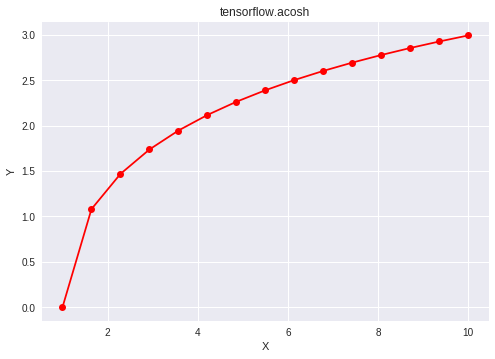Related Articles

# Python | Tensorflow acosh() method

• Last Updated : 10 Dec, 2018

Tensorflow is an open-source machine learning library developed by Google. One of its applications is to develop deep neural networks.

The module `tensorflow.math` provides support for many basic mathematical operations. Function `tf.acosh()` [alias `tf.math.acosh`] provides support for the inverse hyperbolic cosine function in Tensorflow. It expects the input in the range [1, ∞) and returns nan for any input outside this range. The input type is tensor and if the input contains more than one element, element-wise inverse hyperbolic cosine is computed.

Syntax: tf.acosh(x, name=None) or tf.math.acosh(x, name=None)

Parameters:
x: A tensor of any of the following types: float16, float32, float64, complex64, or complex128.
name (optional): The name for the operation.

Return type: A tensor with the same type as that of x.

Code #1:

 `# Importing the Tensorflow library``import` `tensorflow as tf``  ` `# A constant vector of size 6``a ``=` `tf.constant([``1.0``, ``0.5``, ``3.4``, ``-``2.1``, ``0.0``, ``6.5``],``                             ``dtype ``=` `tf.float32)``  ` `# Applying the acosh function and``# storing the result in 'b'``b ``=` `tf.acosh(a, name ``=``'acosh'``)``  ` `# Initiating a Tensorflow session``with tf.Session() as sess:``    ``print``(``'Input type:'``, a)``    ``print``(``'Input:'``, sess.run(a))``    ``print``(``'Return type:'``, b)``    ``print``(``'Output:'``, sess.run(b))`

Output:

```Input type: Tensor("Const:0", shape=(6, ), dtype=float32)
Input: [ 1.   0.5  3.4 -2.1  0.   6.5]
Return type: Tensor("acosh:0", shape=(6, ), dtype=float32)
Output: [0.            nan 1.894559      nan      nan 2.558979]
```

Code #2: Visualization

 `# Importing the Tensorflow library``import` `tensorflow as tf``  ` `# Importing the NumPy library``import` `numpy as np``  ` `# Importing the matplotlib.pylot function``import` `matplotlib.pyplot as plt``  ` `# A vector of size 15 with values from 1 to 10``a ``=` `np.linspace(``1``, ``10``, ``15``)``  ` `# Applying the inverse hyperbolic cosine``# function and storing the result in 'b'``b ``=` `tf.acosh(a, name ``=``'acosh'``)``  ` `# Initiating a Tensorflow session``with tf.Session() as sess:``    ``print``(``'Input:'``, a)``    ``print``(``'Output:'``, sess.run(b))``    ``plt.plot(a, sess.run(b), color ``=` `'red'``, marker ``=` `"o"``) ``    ``plt.title(``"tensorflow.acosh"``) ``    ``plt.xlabel(``"X"``) ``    ``plt.ylabel(``"Y"``) ``  ` `    ``plt.show()`

Output:

```Input: [ 1.          1.64285714  2.28571429  2.92857143  3.57142857  4.21428571
4.85714286  5.5         6.14285714  6.78571429  7.42857143  8.07142857
8.71428571  9.35714286 10.        ]
Output: [0.         1.08055227 1.46812101 1.73714862 1.94591015 2.11724401
2.26282815 2.38952643 2.50174512 2.60249262 2.69391933 2.77761797
2.85480239 2.92641956 2.99322285]
```Attention geek! Strengthen your foundations with the Python Programming Foundation Course and learn the basics.

To begin with, your interview preparations Enhance your Data Structures concepts with the Python DS Course. And to begin with your Machine Learning Journey, join the Machine Learning – Basic Level Course

My Personal Notes arrow_drop_up